Courses

# Vehicle Arrival Models: Count Civil Engineering (CE) Notes | EduRev

## Civil Engineering (CE): Vehicle Arrival Models: Count Civil Engineering (CE) Notes | EduRev

The document Vehicle Arrival Models: Count Civil Engineering (CE) Notes | EduRev is a part of the Civil Engineering (CE) Course Transportation Engineering.
All you need of Civil Engineering (CE) at this link: Civil Engineering (CE)

Introduction

As already noted in the previous chapter that vehicle arrivals can be modelled in two interrelated ways; namely modelling how many vehicle arrive in a given interval of time, or modelling what is the time interval between the successive arrival of vehicles. Having discussed in detail the former approach in the previous chapter, the first part of this chapter discuss how a discrete distribution can be used to model the vehicle arrival. Traditionally, Poisson distribution is used to model the random process, the number of vehicles arriving a given time period. The second part will discuss methodologies to generate random vehicle arrivals, be it the generation of random headways or random number of vehicles in a given duration. The third part will elaborate various ways of evaluating the performance of a distribution.

Poisson Distribution

Suppose, if we plot the arrival of vehicles at a section as dot in a time axis, it may look like Figure 13:1. Let h1, h2, ... etc indicate the headways, then as mentioned earlier, they take some real values. Hence, these headways or inter arrival time can be modelled using some continuous distribution. Also, let t1, t2, tand t4 are four equal time intervals, then the number of vehicles arrived in each of these interval is an integer value. For example, in Fig. 13:1, 3, 2, 3 and 1 vehicles arrived in time interval t1, t2, t3 and t4 respectively. Any discrete distribution that best fit the observed number of vehicle arrival in a given time interval can be used. Similarly, any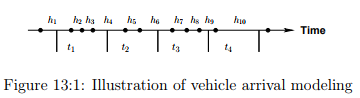continuous distribution that best fit the observed headways (or inter-arrival time) can be used in modelling. However, since these process are inter-related, the distributions that describe these relations should also be inter-related for better explanation of the phenomenon. Interestingly, there exist distributions that meet the above requirements. First, we will see the distribution to model the number of vehicles arrived in a given duration of time. Poisson distribution is commonly used to describe such a random process. The probability density function of the Poisson distribution is given as: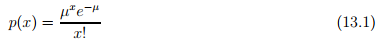where p(x) is the probability for x events will occur in the time interval, and µ is the expected rate of occurrence of that event in that interval. Some special cases of this distribution is given below.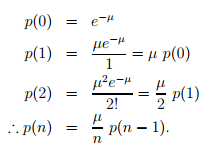Since the events are discrete, the probability that certain number of vehicles (n) arriving in an interval can be computed as: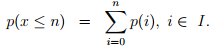Similarly, the probability that the number of vehicles arriving in the interval is exactly in a range (between a and b, both inclusive and a < b) is given as: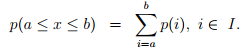Numerical Example

The hourly flow rate in a road section is 120 vph. Use Poisson distribution to model this vehicle arrival.

Solution: The flow rate is given as (µ) = 120 vph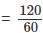= 2 vehicle per minute. Hence, the probability of zero vehicles arriving in one minute p(0) can be computed as follows: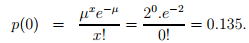Table 13:1: Probability values of vehicle arrivals computed using Poisson distribution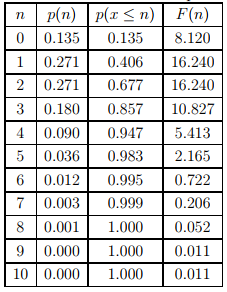Similarly, the probability of one vehicles arriving in one minute p(1) is given by,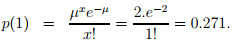Now, the probability that number of vehicles arriving is less than or equal to zero is given as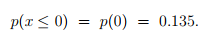Similarly, probability that the number of vehicles arriving is less than or equal to 1 is given as: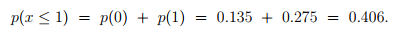Again, the probability that the number of vehicles arriving is between 2 to 4 is given as: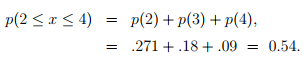Now, if the p(0) = 0.135, then the number of intervals in an hour where there is no vehicle arriving is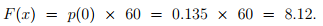The above calculations can be repeated for all the cases as tabulated in Table 13:1. The shape of this distribution can be seen from Figure 13:2 and the corresponding cumulative distribution is shown in Figure 13:3.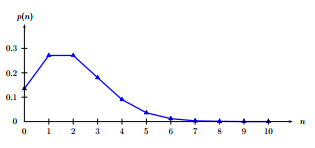Figure 13:2: Probability values of vehicle arrivals computed using Poisson distribution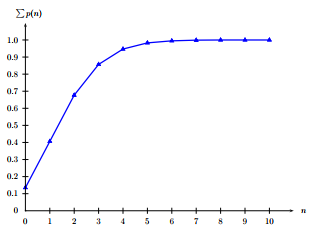Figure 13:3: Cumulative probability values of vehicle arrivals computed using Poisson distribution

Random variates following Poisson distribution

For simulation purposes, it may be required to generate number of vehicles arrived in a given interval so that it follows typical vehicle arrival. This is the reverse of computing the probabilities as seen above. The following steps give the procedure:

1. Input: mean arrival rate µ in an interval t

2. Compute p(x = n) and p(x ≤ n)

3. Generate a random number X such that 0 ≤ X ≤ 1

4. Find n such that p(x ≤ n − 1) ≤ X and p(x ≤ n) ≥ X

5. Set ni = n, where nis the number of vehicles arrived in ith interval.

The steps 3 to 5 can be repeated for required number of intervals.

Numerical Example

Generate vehicles for ten minutes if the flow rate is 120 vph.

Solution The first two steps of this problem is same as the example problem solved earlier and the resulted from the table is used. For the first interval, the random number (X) generated is 0.201 which is greater than p(0) but less than p(1). Hence, the number of vehicles generated in this interval is one (ni = 1). Similarly, for the subsequent intervals. It can also be computed that at the end of 10th interval (one minute), total 23 vehicle are generated. Note: This amounts to 2.3 vehicles per minute which is higher than given flow rate. However, this discrepancy is because of the small number of intervals conducted. If this is continued for one hour, then this average will be about 1.78 and if continued for then this average will be close to 2.02.

Random variates following Exponential distribution

One can generate random variate following negative exponential distribution rather simply due to availability of closed form solutions. The method for generating exponential variates is based on inverse transform sampling:

t = f −1 (X)

Table 13:2: Vehicles generated using Poisson distribution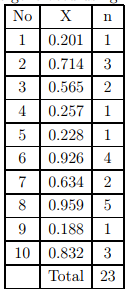has an exponential distribution, where f −1 , called as quantile function, is defined as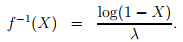Note that if X is uniform, then 1 − X is also uniform and λ = 1/µ. Hence, one can generate exponential variates as follows:

t = −µ × log(X)

where, X is a random number between 0 and 1, µ is the mean headway, and the resultant headways generated (t) will follow exponential distribution.

Numerical Example

Simulate the headways for 10 vehicles if the flow rate is 120 vph. Solution Since the given flow rate is 120 vph, then the mean headway (µ) is 30 seconds. Generate a random number between 0 and 1 and let this be 0.62. Hence, by the above equation, t = 30 × (− log(0.62)) = 14.57. Similarly, headways can be generated. The table below given the generation of 15 vehicles and it takes little over 10 minutes. In other words, the table below gives the vehicles generated for 10 minutes. Note: The mean headway obtained from this 15 headways is about 43 seconds; much higher than the given value of 30 seconds. Of, course this is due to the lower sample size. For example, if the generation is continued to 100 vehicles,

Table 13:3: Headways generated using Exponential distribution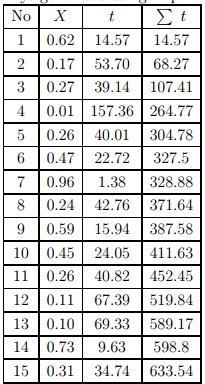then the mean would be about 35 seconds, and if continued till 1000 vehicles, then the mean would be about 30.8 seconds.

Evaluation of the mathematical distribution

The mathematical distribution such as negative exponential distribution, normal distribution, etc needs to be evaluated to see how best these distributions fits the observed data. It can be evaluated by comparing some aggregate statistics as discussed below.

Mean and Standard deviation

One of the easiest ways to compute the mean and standard deviation of the observed data and compare with mean and standard deviation obtained from the computed frequencies. If p c i is the computed probability of the headway is the ith interval, and N is the total number of observations, then the computed frequency of the ith interval is given as: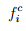=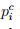× N.

Then the mean of the computed frequencies (µ c ) is obtained as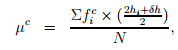where hi is the lower limit of the i th interval, and δh is the interval range. The standard deviation σc can be obtained by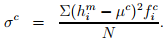If the distribution fit closely, then the mean and the standard deviation of the observed and fitted data will match. However, it is possible, that two sample can have similar mean and standard deviation, but, may differ widely in the individual interval. Hence, this can be considered as a quick test for the comparison purposes. For better comparison, Chi-square test which gives a better description of the suitability of the distribution may be used.

Chi-square test

The Chi-square value (X2 ) can be computed using the following formula: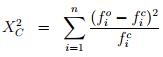where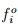is the observed frequency,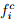is the computed (theoretical) frequency of the i th interval, and n is the number of intervals. Obviously, a X2 value close to zero implies a good fit of the data, while, high X2 value indicate poor fit. For an objective comparison Chi-square tables are used. A chi-square table gives X2 values for various degree of freedom. The degree of freedom (DOF) is given as

DOF = n − 1 − p

where n is the number of intervals, and p is the number of parameter defining the distribution. Since negative exponential distribution is defined by mean headway alone, the value of p is one, where as Pearson and Normal distribution has the value of p as two, since they are defined by µ and σ. Chi-square value is obtained from various significant levels. For example, a significance level of 0.05 implies that the likelihood that the observed frequencies following the theoretical distribution is is 5%. In other words, one could say with 95% confidence that the observed data follows the theoretical distribution under testing.

Numerical Example

Compute the X2 statistic of the following distribution, where N = 2434.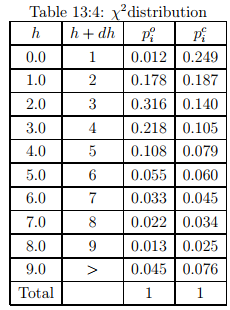Solution: The given headway range and the observed probability is given in column (2), (3) and (4). The observed frequency for the first interval (0 to 1) can be computed as the product of observed probability pi and the number of observation (N) i.e.=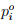× N = 0.012 × 2434 = 29.21 as shown in column (5). Now the computed frequency for the first interval (0 to 1) is the product of computed probability and the number of observation (N) i.e.=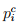×N = 0.249×2434 = 441.21 as shown in column (7). The χ value can be computed as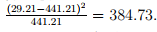Similarly, all the rows are computed and the total χ 2 value is obtained as 1825.52. A chi-square table gives Xvalues for various degree of freedom. The degree of freedom (DOF) is given as: DOF = n − 1 − p = 10 − 1 − 1 = 8, where n is the number of intervals (10), and p is the number of parameter (1 because it is exponential distribution). Now at a significance level of 0.05 and DOF 8, from the table,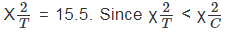hence reject that the observed frequency follows exponential distribution.

Conclusion

The chapter covers three aspects: modeling vehicle arrival using Poisson distribution, generation of random variates following certain distribution, and evaluation of distributions. Specific evaluation include comparing the mean and standard deviation at macro level and using chisquare test which is essentially a micro-level comparison.

The document Vehicle Arrival Models: Count Civil Engineering (CE) Notes | EduRev is a part of the Civil Engineering (CE) Course Transportation Engineering.
All you need of Civil Engineering (CE) at this link: Civil Engineering (CE)Use Code STAYHOME200 and get INR 200 additional OFF Use Coupon Code
All Tests, Videos & Notes of Civil Engineering (CE): Civil Engineering (CE)

### Top Courses for Civil Engineering (CE)## Transportation Engineering

23 videos|66 docs|39 tests

### Top Courses for Civil Engineering (CE)Track your progress, build streaks, highlight & save important lessons and more!

,

,

,

,

,

,

,

,

,

,

,

,

,

,

,

,

,

,

,

,

,

;